Superconductivity

This free course is available to start right now. Review the full course description and key learning outcomes and create an account and enrol if you want a free statement of participation.

Free course

4.2 Type-I superconductors

You saw in Subsection 2.4 that superconductivity in a tin cylinder is destroyed when an applied field with strength B﻿0  > B﻿c is applied parallel to the cylinder. However, when the field is applied perpendicular to the cylinder, as shown in Figure 18, the field strength at points A and C is substantially greater than the strength of the applied field at a distance from the cylinder, and this is indicated by the increased concentration of the field lines shown near these points. In fact, it can be shown that the field strength at these points is a factor of two greater than the applied field strength. This means that as the applied field B﻿0 is increased, the field at points A and C will reach the critical field strength Bc when B0 = B﻿c﻿/﻿2.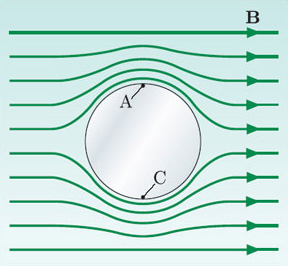Figure 18 The magnetic field B in a plane perpendicular to the axis of a superconducting cylinder (shown in cross-section) for an applied field with B﻿0 < B﻿c﻿/﻿2.

You might think that superconductivity in the cylinder would be completely destroyed at this lower field strength. However, were this to be the case, then the material would be in the normal state with a field strength in its interior of less than B﻿c, which is not possible. Instead, for applied field strengths B﻿0 in the range B﻿c﻿/﻿2 < B﻿0 < B﻿c, the cylinder splits up into small slices of normal and superconducting material that run parallel to the applied field. This state in which regions of normal and superconducting material coexist in a type-I superconductor is known as the intermediate state, and it is shown schematically in Figure 19. Within the normal regions, B = B﻿c, and in the superconducting regions, B decreases rapidly and is confined to a thin layer, the width of which is determined by the penetration depth, as shown in Figure 20. The number density of superconducting electrons n﻿s increases from zero at the boundary to the bulk value over the coherence length ξ. Note that since n﻿s is not constant in the superconducting regions, the magnetic field strength does not fall exponentially. The proportion of the material in the normal state increases from zero for B﻿0 = B﻿c﻿/﻿2 to 100 per cent for B﻿0 = B﻿c.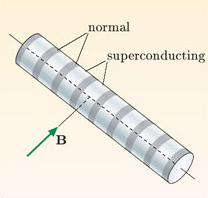Figure 19 Schematic representation of the intermediate state for a cylinder of a type-I superconductor aligned perpendicular to the magnetic field.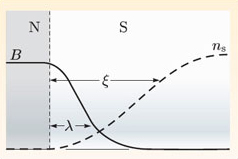Figure 20 Variation of magnetic field strength and number density of superconducting electrons in the region of a boundary between normal and superconducting regions in the intermediate state of a type-I superconductor.

The lowest applied field strength at which the intermediate state appears depends on the shape of the specimen and the orientation of the field. Essentially, it is determined by the extent to which the field is deviated by the superconductor, or equivalently, by how much the field strength is enhanced at the edges of the superconductor. For the thin cylinder shown in Figure 21a, the field just outside is essentially the same as the applied field, so there is a direct transition from superconducting to normal state, without the intervening intermediate state. Contrast this with the thin plate oriented perpendicular to the applied field shown in Figure 21b, where the field strength would be greatly enhanced outside the plate's edges if it could not penetrate the plate. Samples like this enter the intermediate state when the applied field strength is a very small fraction of the critical field strength (Figure 21c).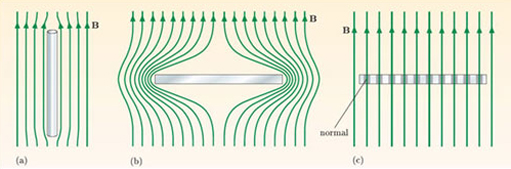Figure 21 (a) A thin superconducting cylinder hardly distorts a uniform applied field. (b) Highly distorted field that would result if a magnetic field did not penetrate a thin plate. (c) Intermediate state in a thin plate.

Figure 22 shows the pattern of normal and superconducting regions for an aluminium plate in the intermediate state, with the field perpendicular to the surface.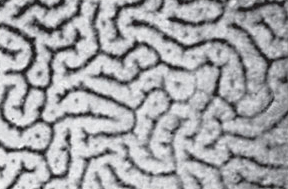Figure 22 Intermediate state in an aluminium plate. Tin powder was deposited on the plate and collected in the superconducting regions – the dark areas of the image – where the magnetic field was low. The light normal regions are about 1 mm wide.

SMT359_1